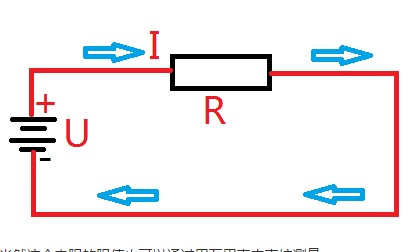# What is the impedance? What is the role of impedance matching?

In circuits with resistors, inductors, and capacitors, the blocking effect on the current in the circuit is called impedance. The impedance is usually represented by Z, which is a complex number. The real part is called a resistor, and the imaginary part is called a reactance. The blocking effect of the capacitor on the alternating current in the circuit is called the capacitive reactance. The inductance acts on the alternating current in the circuit. For inductive reactance, the blocking effect of capacitors and inductors on the AC in the circuit is collectively called reactance. The unit of impedance is ohms. The concept of impedance is not only present in the circuit, but also in the mechanical vibration system.

Impedance is a physical quantity that represents the performance of a component or the electrical performance of a segment of a circuit. The ratio of the peak value (or rms) Um across a passive circuit in an AC circuit to the peak (or rms) of the current through the circuit is called the impedance, expressed in z, in ohms (Ω). In the case where U is constant, the larger z is, the smaller I is, and the impedance has a limiting effect on the current.The importance of impedance matching

The specific relationship between the load impedance and the impedance within the signal during signal transmission. A relationship between the output impedance of a piece of equipment and the connected load impedance, so as to avoid a significant impact on the working state of the equipment itself after the load is connected. For the interconnection of electronic equipment, for example, the signal source is connected to the amplifier, and the front stage is connected to the subsequent stage. As long as the input impedance of the latter stage is greater than 5-10 times of the output impedance of the previous stage, the impedance matching is considered good; for the amplifier connection For the speaker, the tube machine should use a speaker that is equal or close to the nominal impedance of the output, and the transistor amplifier does not have this limitation, and can be connected to any impedance speaker.

Impedance matching has two main functions, adjusting load power and suppressing signal reflection.

Impedance matching method

There are two main methods of impedance matching, one is to change the impedance, and the other is to adjust the transmission line.

Changing the impedance is to adjust the load impedance value through series and parallel connection of capacitor, inductor and load to achieve source and load impedance matching.

Adjusting the transmission line is to lengthen the distance between the source and the load, and adjust the impedance to zero with the capacitance and inductance. At this point, the signal will not be emitted and the energy can be absorbed by the load. In high-speed PCB layout, the trace impedance of the digital signal is generally designed to be 50 ohms. The coaxial cable baseband is generally specified to be 50 ohms, with a frequency band of 75 ohms and a twisted pair (differential) of 85-100 ohms.

Impedance matching application

For general high-frequency signal fields, such as clock signals, bus signals, and even DDR signals up to several hundred megabits, the inductive reactance and capacitive reactance of the transceiver side of the general device are relatively small, and the relative resistance (ie, the real part of the impedance) comes. It can be ignored, in this case, the impedance matching only needs to consider the real part.

In the field of radio frequency, many devices such as antennas, power amplifiers, etc. whose input and output impedances are non-real (non-pure resistors), and their imaginary parts (capacitive reactance or inductive reactance) are so large that they cannot be ignored. In this case, conjugate matching is used. Methods.